Next: Electric Current Up: Capacitance Previous: Example 6.3: Equivalent capacitance

## Example 6.4: Energy stored in a capacitor

Question: An air-filled parallel plate capacitor has a capacitance of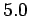pF. A potential of 100V is applied across the plates, which are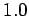cm apart, using a storage battery. What is the energy stored in the capacitor? Suppose that the battery is disconnected, and the plates are moved until they are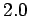cm apart. What now is the energy stored in the capacitor? Suppose, instead, that the battery is left connected, and the plates are again moved until they arecm apart. What is the energy stored in the capacitor in this case?

Answer: The initial energy stored in the capacitor is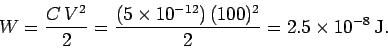When the spacing between the plates is doubled, the capacitance of the capacitor is halved to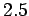pF. If the battery is disconnected then this process takes place at constant charge. Thus, it follows from the formula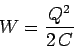that the energy stored in the capacitor doubles. So, the new energy is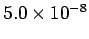J. Incidentally, the increased energy of the capacitor is accounted for by the work done in pulling the capacitor plates apart (since these plates are oppositely charged, they attract one another).

If the battery is left connected, then the capacitance is still halved, but now the process takes place at constant voltage. It follows from the formula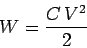that the energy stored in the capacitor is halved. So, the new energy is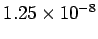J. Incidentally, the energy lost by the capacitor is given to the battery (in effect, it goes to re-charging the battery). Likewise, the work done in pulling the plates apart is also given to the battery.Next: Electric Current Up: Capacitance Previous: Example 6.3: Equivalent capacitance
Richard Fitzpatrick 2007-07-14